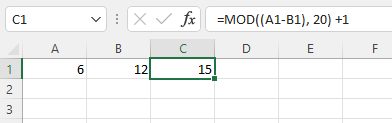# Modulo differences (LibreOffice v PHP)

I am trying to reproduce some spreadsheet formulae in a PHP script.

``````=MOD((D25-D7),20)+1
``````

which I have written in PHP as:

``````(\$D25 - \$D7) % 20 + 1;
``````

With D25 = 6 and D7 = 12 the spreadsheet gives an answer of 15 and the PHP script gives an answer of -5.

I’m puzzled as to how the spreadsheet (I’m using LibreOffice) gets an answer of 15.

Edit: Hopefully Excel would give the same result, but I can’t check.

Excel matches Libre1 Like

You’ve run into a debate as to what mod (%) really is, and whether negative numbers are allowed. Excel defined mod to be: MOD(n, d) = n - d × INT(n/d) and with that int() in there, the question becomes how to round a negative integer. php chose to do it purely on the math of calculating the remainder, which is different.

I just wrote this, so do some testing, but it may work:

``````function mod(\$n,\$d)
{
return \$n - \$d * floor(\$n/\$d);
}``````
1 Like

Libre does the same thing, and it looks like anything over .0 is rounded up for both of them. At that point the math makes sense

``````MOD(x,y) = x - (y * INT(x/y))
MOD(x,y) = (6-12) - (20 * INT((6-12)/20))
MOD(x,y) = -6 - (20 * INT(-6/20))
MOD(x,y) = -6 - (20 * INT(-0.3))
MOD(x,y) = -6 - (20 * -1)
MOD(x,y) = -6 - (-20)
MOD(x,y) = -6 + 20
MOD(x,y) = 14
``````
1 Like

yes, whereas in PHP:

``````echo intval(-0.3);
0``````

Giving you the difference in the final result. That’s why I switched it to floor(), which returns -1 for floor (-0.3);

1 Like

The definition gets a bit ambiguous when dealing with negatives. Different languages deal with it differently, so you will get this disparity in different programs/languages.

2 Likes

Thanks, guys. At least I now know why! I think I vaguely remember this as an issue when I first started programming, more years ago than I care to admit.This topic was automatically closed 91 days after the last reply. New replies are no longer allowed.